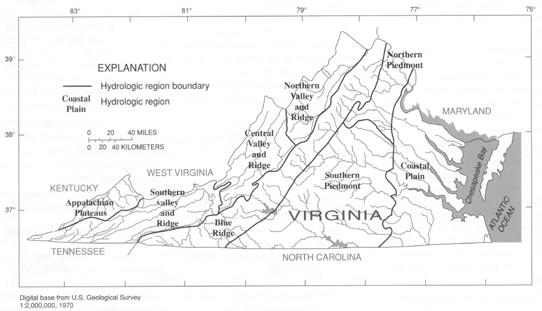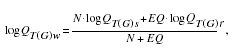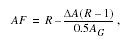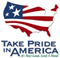# The National Flood-Frequency Program—Methods for Estimating Flood Magnitude and Frequency in Rural Areas in Virginia, 2000

#### U.S. Geological Survey Fact Sheet 023-01 February, 2001

This report is available in pdf 497KB.

## Introduction

Estimates of the magnitude and frequency of flood-peak discharges and flood hydrographs are used for a variety of purposes, such as for the design of bridges, culverts, and flood-control structures; and for the management and regulation of flood plains. To provide simple methods of estimating flood-peak discharges, the U.S. Geological Survey (USGS) has developed and published equations for every State, the Commonwealth of Puerto Rico, American Samoa, and a number of metropolitan areas in the United States. In 1993, the USGS, in cooperation with the Federal Emergency Management Agency and the Federal Highway Administration, compiled all current USGS statewide and metropolitan area equations into a computer program, titled “The National Flood- Frequency (NFF) Program” (Jennings and others, 1994).

Since 1993, new or updated equations have been developed by the USGS for various areas of the Nation. These new equations have been incorporated into an updated version of the NFF Program.

This Fact Sheet describes the application of the updated NFF Program to streams that drain rural areas in Virginia. Information on obtaining the NFF software and fact sheets for other areas of the Nation is provided at the end of this Fact Sheet.

## Overview

Bisese (1995) developed regression equations for estimating peak discharges (QT), in cubic feet per second, at recurrence intervals (T) that range from 2 to 500 years. Separate sets of equations were developed for each of the eight hydrologic regions of Virginia (fig. 1). These regions were defined on the basis of physiography, drainage, and geographic grouping of regression residuals.Figure 1. Hydrologic regions of Virginia.

Recurrence interval is the reciprocal of the annual exceedance probability, and represents the average number of years between exceedances. For example, a flood peak discharge with an exceedance probability of 0.01 has a recurrence interval of 100 years. This does not imply that the 100-year flood will be equaled or exceeded each 100 years, but that it will be equaled or exceeded on the average once every 100 years.

The regression equations were developed using peak-discharge data and watershed characteristics from 276 streamgaging stations in Virginia and 8 streamgaging stations in Kentucky. All stations used to develop the equations had at least 10 years of record between 1885 and 1991. Bisese (1995) summarized the peak-discharge estimates and watershed characteristics for the 284 streamgaging stations used to develop the regression equations, and for 79 additional stations in Virginia.

The regression equations are not applicable to sites affected by channelization, urbanization, or regulation. A drainage basin in which more than 10 percent of the drainage area is impounded was considered regulated.

## Procedure

The equations are based on the inch-pound system of units, but the NFF Program will accept or report results in either the inch-pound or metric system of units. The explanatory watershed variables used in the regression equations are as follows:

Drainage area (A), in square miles, is the total area that contributes runoff upstream of the stream site of interest and is determined from either 1:24,000- or 1:62,500-scale topographic maps.

Main channel length (L), in miles, is the distance along the main channel from the stream site of interest to the drainage divide and is determined from the same maps as for drainage area. At stream junctions, the branch that drains the largest area is considered the main channel.

Main channel slope (Sl), in feet per mile, is the difference in elevations at points 10 and 85 percent of the distance along the main channel (measured from the location of the desired peak discharge to the drainage divide), divided by the distance between the points.

Mean basin elevation (E), in feet above sea level [National Geodetic Vertical Datum of 1929 (NGVD of 1929)], is the mean elevation of the drainage basin determined from 1:250,000-scale topographic maps by the grid-sampling technique. A transparent grid is placed over the map on which the basin boundary is drawn. The elevation is determined for at least 20 grid intersections within the basin, and the values are averaged.

Area of forest (F), is the percentage of drainage area, A, that is covered by forest. Forest areas were determined from USGS 1:250,000-scale quadrangle maps by measuring areas with green overprint. A constant of 1 is added to F by the NFF program; the user enters the actual value of F.

The regression equations, the average standard errors of prediction, and the equivalent years of record are shown in table 1. The average standard error of prediction is a measure of the accuracy of an equation when used to estimate peak discharges for ungaged watersheds similar to those that were used to derive the regression equations. Errors in the estimates for about two-thirds of the ungaged sites will be within the given standard errors. The average standard error of prediction increases appreciably when any explanatory watershed variable is near or outside the quoted range. The ranges of the explanatory watershed variables for which the equations are applicable are shown in table 2.

 Regression equation Average Equivalent standard years of error of record prediction, in percent Table 1. Flood-peak discharge regression equations and associated statistics for streams that drain rural areas in Virginia (modified from Bisese, 1995) [QT, peak discharge for recurrence interval T, 2 to 500 years, in cubic feet per second; A, drainage area, in square miles; Sl, main channel slope, in feet per mile; L, main channel length, in miles; E, mean basin elevation, in feet above sea level (NGVD of 1929); F, area of forest, in percent] Coastal Plain Q2 = 2.4A1.005Sl0.852 57.1 1.4 Q5 = 4.0A0.999Sl0.884 59.7 2.5 Q10 = 4.9A1.005Sl0.932 59.4 3.8 Q25 = 6.0A1.016Sl0.998 61.0 5.6 Q50 = 6.8A1.024Sl1.044 64.1 6.7 Q100 = 7.6A1.033Sl1.088 68.5 7.5 Q200 = 8.3A1.042Sl1.130 73.9 8.0 Q500 = 9.2A1.055Sl1.185 82.7 8.5 Northern Piedmont Q2 = 179A0.655 51.1 1.6 Q5 = 317A0.644 49.3 3.3 Q10 = 438A0.641 50.2 4.9 Q25 = 626A0.640 53.8 6.7 Q50 = 793A0.640 58.0 7.7 Q100 = 984A0.641 63.5 8.2 Q200 = 1,200A0.643 70.1 8.5 Q500 = 1,535A0.646 80.4 8.6 Southern Piedmont Q2 = 21.6A0.881E0.310L-0.423 40.2 2.8 Q5 = 31.9A0.854E0.351L-0.417 35.7 6.2 Q10 = 38.8A0.848E0.379L-0.430 35.5 9.3 Q25 = 54.8A0.852E0.392L-0.463 38.0 12.3 Q50 = 74.3A0.860E0.390L-0.495 41.4 13.6 Q100 = 101A0.869E0.382L-0.529 45.7 14.2 Q200 = 136A0.879E0.373L-0.561 50.6 14.4 Q500 = 197A0.893E0.361L-0.602 58.0 14.2 Blue Ridge Q2 = 95.4A0.760 33.4 4.0 Q5 = 201A0.726 34.1 6.5 Q10 = 298A0.710 35.5 8.8 Q25 = 450A0.695 38.8 11.0 Q50 = 584A0.687 42.2 12.0 Q100 = 735A0.680 46.2 12.5 Q200 = 907A0.674 50.7 12.6 Q500 = 1,165A0.667 56.7 12.8 Northern Valley and Ridge Q2 = 73.0A0.955L-0.307(F+1)0.041 37.8 3 .6 Q5 = 119A0.953L-0.290(F+1)0.063 33.5 7 .4 Q10 = 153A0.944L-0.273(F+1)0.081 31.4 12 .2 Q25 = 196A0.931L-0.251(F+1)0.107 30.9 18 .5 Q50 = 228A0.926L-0.241(F+1)0.124 31.9 22 .2 Q100 = 263A0.925L-0.237(F+1)0.138 33.8 24 .4 Q200 = 300A0.928L-0.239(F+1)0.149 36.3 25 .3 Q500 = 356A0.936L-0.247(F+1)0.161 40.8 25 .1 Central Valley and Ridge Q2 = 89.2A0.788 31.0 4 .8 Q5 = 222A0.712 29.3 8 .7 Q10 = 372A0.668 28.6 12 .9 Q25 = 647A0.620 29.5 17 .5 Q50 = 918A0.591 31.4 19 .4 Q100 = 1,254A0.565 34.1 20 .2 Q200 = 1,665A0.542 37.4 20 .2 Q500 = 2,354A0.514 42.6 19 .5 Southern Valley and Ridge Q2 = 45.7A0.880 45.0 1 .7 Q5 = 89.5A0.825 43.4 2 .6 Q10 = 127A0.800 44.2 3 .3 Q25 = 181A0.774 46.6 4 .2 Q50 = 228A0.759 49.1 4 .7 Q100 = 281A0.745 52.0 5 .2 Q200 = 339A0.733 55.3 5 .5 Q500 = 425A0.718 60.2 5 .7 Appalachian Plateaus Q2 = 262A0.749Sl-0.175 33.6 3 .5 Q5 = 134A0.844Sl0.032 21.3 12 .2 Q10 = 103A0.880Sl0.136 18.1 23 .5 Q25 = 90.4A0.902Sl0.227 19.3 31 .5 Q50 = 87.0A0.910Sl0.280 21.9 33 .0 Q100 = 85.7A0.916Sl0.324 24.7 33 .4 Q200 = 85.0A0.920Sl0.365 27.9 33 .5 Q500= 85.5A0.923Sl0.411 31.9 33 .5
 Hydrologic study Drainagearea, in square miles Main Main Average Area of region channel channel length, basin forest, in percent slope, in miles elevation, in feet in feet per mile above sea level1 Table 2. Range of explanatory variables for which regression equations are applicable (modified from Bisese, 1995) Coastal Plain—29 Sites 0.7–617 1.6–83 1.2–65 20–260 0–94 Northern Piedmont—19 Sites 0.1–570 6.3–400 0.3–54 310–465 39–96 Southern Piedmont—67 Sites 0.3–2,730 2.6–173 0.7–184 80–1,100 16–98 Blue Ridge—54 Sites 0.6–1,340 7.8–880 1.0–131 560–3,280 16–99 Northern Valley and Ridge—29 Sites 0.3–1,642 7.7–973 1.0–145 760–3,330 1–99 Central Valley and Ridge—34 Sites 0.7–3,259 9.3–592 1.7–169 1,280–2,890 20–98 Southern Valley and Ridge—35 Sites 1.2–672 9.7–324 2.2–124 1,500–2,810 4–95 AppalachianPlateaus—17 Sites 0.7–554 10.2–510 1.3–53 1,450–2,500 0–96 1NGVD of 1929.

## Improving Estimates With Gaged Data

The U.S. Water Resources Council (1981, appendix 8) described weighting techniques to improve estimates of peak discharge at gaged locations by combining the estimates derived from analysis of gage records with estimates derived from other means, including regression equations. The weights of the two independent estimates are based on the length of the gage record (in years) and the equivalent years of record of the applicable regression equation. The weighted estimate of peak discharge is computed as:where

 QT(G)w is the weighted estimate of discharge Q for recurrence interval T at the gage location, QT(G)s is the estimate of QT derived from analysis of the systematic gage records, QT(G)r is the estimate of QT derived from application of the appropriate regression equation in table 1, N is the number of years in the gage record used to compute QT(G)s, and EQ is the equivalent years of record (table 1).

The accuracy of the weighted discharge estimate, in equivalent years of record, is equal to N + EQ. The NFF Program contains an algorithm to perform these computations, which differs slightly from the computations described by Bisese (1995).

## Ungaged Sites Near Gaged Sites on the Same Stream

Bisese (1995) showed how the weighted estimate for peak discharge at a gaged site can be used to improve estimates of peak discharge at an ungaged site on the same stream that has a drainage area that is between 50 and 150 percent of the drainage area of the gaged site. The regression estimate for the ungaged site is multiplied by an adjustment factor, which is computed as:where

 AF is the adjustment factor, ΔA is the absolute value of difference in drainage area between the gaged site (AG) and the ungaged site (AU), |AG -AU|, and R is the ratio of the weighted peak-discharge estimate to the regression estimate for the gaged site, QT(G)w/QT(G)r.

The adjustment to the weighted estimate at the gage site can be used when the drainage area at the ungaged site is within 50 to 150 percent of the drainage area at the gaged site. Otherwise, the estimate at the ungaged site should be based only on the appropriate regression equation.

## Sites in Transition Zones

When the drainage area of the site of interest is in more than one region, a weighted estimate of the peak discharge should be computed. The equations for the appropriate regions should be applied independently using basinwide estimates of the required explanatory variables. The weighted estimate is then computed by multiplying each regional estimate against the fraction of the drainage area in that region and summing the products. The NFF Program provides an algorithm for this computation.

## References

Bisese, J.A., 1995, Methods for estimating the magnitude and frequency of peak discharges of rural, unregulated streams in Virginia: U.S. Geological Survey Water-Resources Investigations Report 94–4148, 70 p.

Jennings, M.E., Thomas, W.O., Jr., and Riggs, H.C., comps., 1994, Nationwide summary of U.S. Geological Survey regional regression equations for estimating magnitude and frequency of floods for ungaged sites, 1993: U.S. Geological Survey Water-Resources Investigations Report 94–4002, 196 p.

U.S. Water Resources Council, 1981, Guidelines for determining flood flow frequency: U.S. Water Resources Council Bulletin 17B, 28p. 14 appendixes.

For more information contact:

U.S. Geological Survey
Office of Surface Water
415 National Center
Reston, Virginia 20192
(703) 648-5301

USGS hydrologic analysis software is available for electronic retrieval through the World Wide Web (WWW) at: http://water.usgs.gov/software/ and through anonymous File Transfer Protocol (FTP) from water.usgs.gov (directory: /pub/software). The WWW page and FTP directory from which the National Flood-Frequency software and user documentation can be retrieved are http://water.usgs.gov/software/nff.html and /pub/software/surface_water/nff, respectively.

Additional earth science information is available from the USGS through the WWW at http://www.usgs.gov/ or by calling 1-888-ASK-USGS.

 AccessibilityFOIAPrivacyPolicies and Notices U.S. Department of the Interior, U.S. Geological Survey Persistent URL: Page Contact Information: Contact USGS Last modified: Tuesday, November 29 2016, 05:53:29 PM